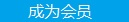您可以捐助，支持我们的公益事业。 1元 10元 50元 认证码：必填求知 文章 文库 Lib 视频 iProcess 课程 认证 咨询 工具 讲座 Modeler Code要资料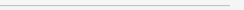订阅捐助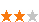919 次浏览     评价： 好 中 差
2018-11-22

 编辑推荐: 本文来自于csdn，本文章主要介绍了真实数据（csv表格数据）训练集的查看与预处理以及Pineline的基本架构的实战操作，希望对您的学习有帮助。

7、选择及训练模型

 from sklearn.linear_model import LinearRegression lin_reg = LinearRegression() lin_reg.fit(train_housing_prepared, train_housing_labels)

 from sklearn.metrics import mean_squared_error housing_predictions = lin_reg.predict(train_housing_prepared) lin_mse = mean_squared_error(train_housing_labels, housing_predictions) lin_rmse = np.sqrt(lin_mse) lin_rmse

 from sklearn.tree import DecisionTreeRegressor tree_reg = DecisionTreeRegressor() tree_reg.fit(train_housing_prepared, train_housing_labels) housing_predictions = tree_reg.predict(train_housing_prepared) tree_mse = mean_squared_error(train_housing_labels, housing_predictions) tree_rmse = np.sqrt(tree_mse) tree_rmse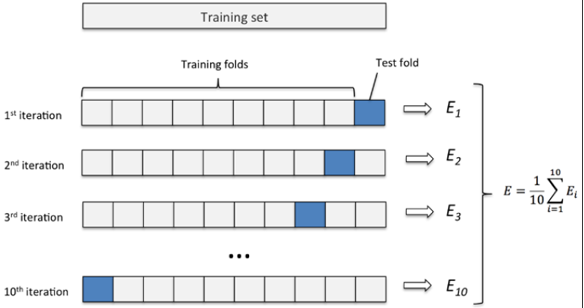from sklearn.model_selection import cross_val_score tree_scores = cross_val_score(tree_reg, train_housing_prepared, train_housing_labels, scoring="neg_mean_squared_error", cv=10) lin_scores = cross_val_score(lin_reg, train_housing_prepared, train_housing_labels, scoring="neg_mean_squared_error", cv=10) tree_rmse_scores = np.sqrt(-tree_scores) lin_rmse_scores = np.sqrt(-lin_scores) def display_scores(scores): print("Scores:", scores) print("Mean:", scores.mean()) print("Standard deviation:", scores.std()) display_scores(tree_rmse_scores) display_scores(lin_rmse_scores)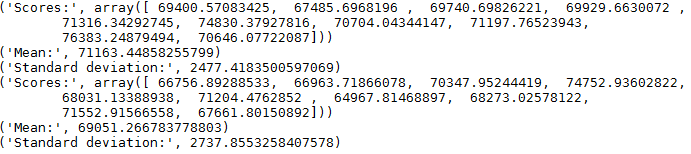from sklearn.externals import joblib joblib.dump(my_model, "my_model.pkl") #保存模型 # and later... my_model_loaded = joblib.load("my_model.pkl") #加载模型

8、模型调参

scikit-learn中提供函数GridSearchCV用于网格搜索调参，网格搜索就是通过自己对模型需要调整的几个参数设定一些可行值，然后Grid Search会排列组合这些参数值，每一种情况都去训练一个模型，经过交叉验证今后输出结果。下面为随机森林回归模型（RandomForestRegression）的一个Grid Search的例子。

 from sklearn.model_selection import GridSearchCV param_grid = [ {'n_estimators': [3, 10, 30], 'max_features': [2, 4, 6, 8]}, {'bootstrap': [False], 'n_estimators': [3, 10], 'max_features': [2, 3, 4]}, ] forest_reg = RandomForestRegressor() grid_search = GridSearchCV(forest_reg, param_grid, cv=5, scoring='neg_mean_squared_error') grid_search.fit(train_housing_prepared, train_housing_labels)

 grid_search.best_params_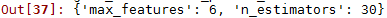grid_search.best_params_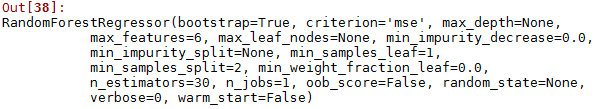cvres = grid_search.cv_results_ ... for mean_score, params in zip(cvres["mean_test_score"], cvres["params"]): ... print(np.sqrt(-mean_score), params)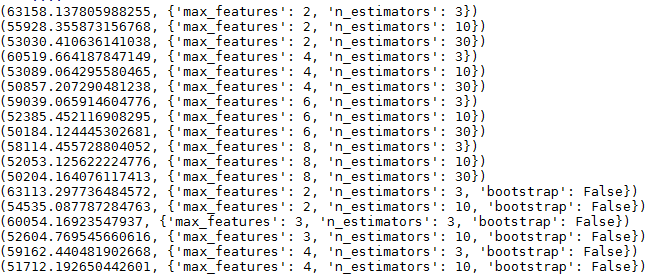from sklearn.model_selection import RandomizedSearchCV param_ran={'n_estimators':range(30,50),'max_features': range(3,8)} forest_reg = RandomForestRegressor() random_search = RandomizedSearchCV(forest_reg,param_ran,cv=5, scoring='neg_mean_squared_error',n_iter=10) random_search.fit(train_housing_prepared, train_housing_labels)

 feature_importances = grid_search.best_estimator_.feature_importances_ extra_attribs = ["rooms_per_hhold", "pop_per_hhold", "bedrooms_per_room"] cat_one_hot_attribs = list(encoder.classes_) attributes = num_attribs + extra_attribs + cat_one_hot_attribs sorted(zip(feature_importances, attributes), reverse=True)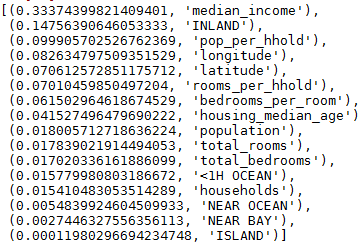final_model = grid_search.best_estimator_ X_test = strat_test_set.drop("median_house_value", axis=1) y_test = strat_test_set["median_house_value"].copy() X_test_prepared = full_pipeline.transform(X_test) final_predictions = final_model.predict(X_test_prepared) final_mse = mean_squared_error(y_test, final_predictions) final_rmse = np.sqrt(final_mse)919 次浏览  评价: 好 中 差订阅捐助
 相关文章 我们该如何设计数据库 数据库设计经验谈 数据库设计过程 数据库编程总结
 相关文档 数据库性能调优技巧 数据库性能调整 数据库性能优化讲座 数据库系统性能调优系列
 相关课程 高性能数据库设计与优化 高级数据库架构师 数据仓库和数据挖掘技术 Hadoop原理、部署与性能调优每天2个文档/视频 扫描微信二维码订阅
 订阅技术月刊 获得每月300个技术资源